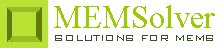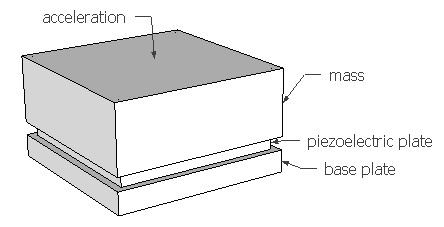PIZOACEL,pcoef,area,thc,m,acel,freq,rper,ymod,sel

pcoef     longitudinal piezoelectric coefficient in C/m2
area      area of the electrode or piezo plate in µm2
thc         thickness of the piezoelectric element in µm
m           inertial mass load in kg
acel       applied acceleration in g
freq       signal frequency in Hz
rper       relative permittivity of the piezoelectric material
ymod    Young's modulus of the material in GPa
sel         number denoting the selected result.
Use 1 for output current, 2 for output voltage and 3 for piezoelement capacitanceNotes

Some materials generate an electric field when subjected to mechanical deformation under stress. This effect is called piezoelectric effect. Piezoelectric sensing offers some advantages over other forms like capacitive and piezoresistive sensing. It is self generating and so requires no bias voltage or current. Its ability to sense and actuate is especially advantageous for use in resonators. But piezoelectric sensing can be used only to monitor a change in signal and cannot detect a static signal. So an accelerometer based on piezoelectric effect cannot sense a static acceleration but can sense a change in acceleration.

When the applied stress and resulting strain are parallel to the electric field, the sensing element is described to be in longitudinal configuration. The accelerometer setup shown in the picture above is in longitudinal configuration. The setup is similar to commercially available accelerometers. The stress is generated by the inertial mass pushing against the piezoelectric material under a sinusoidal acceleration signal. The piezoelectric coefficient that couples the stress and electric field is the longitudinal piezoelectric coefficient in this case. The piezoelectric coefficients for some common piezoelectric materials can be found under the material properties section.

The motional current generated by the sensor is proportional to the rate of change of the signal or strain. The current can be measured using a voltage amplifier.  The motional current is converted to voltage by the piezoelement capacitance in parallel with the circuit impedances. For calculating the output voltage, it is assumed that the element capacitance dominates the total capacitance and the signal frequency is above the -3dB cut-off frequency.

The plot shows the frequency response of the sensor on a logarithmic scale. It gives the output current as a function of the signal frequency.

Assumptions

-The strain in the transducer is assumed to be uniform.
-The strains and electric fields in non-longitudinal directions are assumed to be zero.
-The input frequency is assumed to be well above the -3dB cut-off frequency of the signal conditioning circuit.
-The piezoelectric element capacitance is well above the circuit impedances.